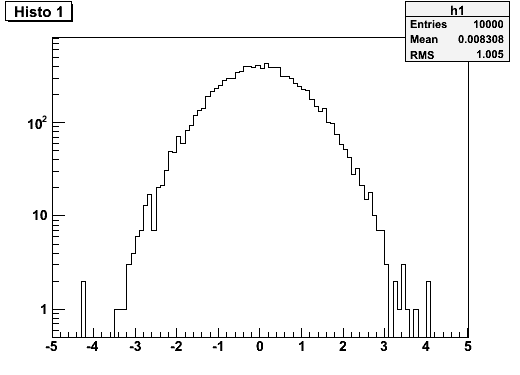# Axis labels in log scale

Is there a way to make all axis labels “exponent-iated” if the axis is set to logscale?

To clarify, I’d like to have linear x axis and logarithmic y axis, with the latter ranging from e.g. 0.1 to 1000. However, rather than printing “1” and “10” I prefer 10^0 and 10^1.

Cheers,

Maurits

What you are asking is the default behavior. Example:

``````{
TCanvas *c1 = new TCanvas("c1","c1",800,600);
c1->SetLogy();
TH1F *h1 = new TH1F("h1","Histo 1",100,-5,5);
h1->FillRandom("gaus",10000);
h1->Draw();
}``````By the way it is not possible to get 10^0 and 10^1 .
The notation with power of 10 start at 10^2.
We will not implement 10^0 and 10^1.

Hi couet.

Thanks for clearing that up. I was very well aware of the default behaviour.
However, I still prefer 10^0 and 10^1 rather than 1 and 10, and in my opinion former notation is much more consistent.
As I like to avoid (or minimize) the amount of post-processing of ROOT plots, it would have been good if there was that option.

Cheers,

Maurits

We do not think 10^0 and 10^1 are the common way to draw log scales. It looks really ugly. The 0 et 1 exponents are removed on purpose.